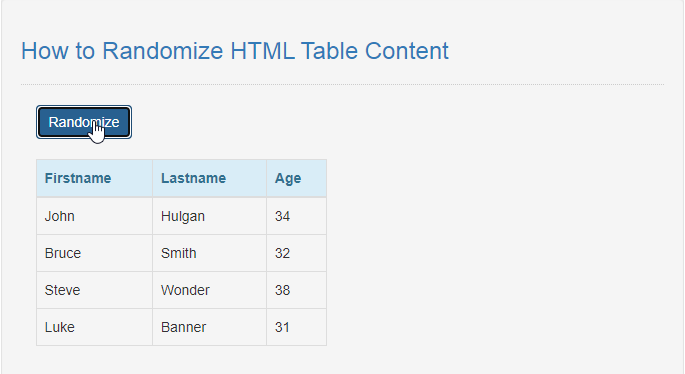# How to Randomize HTML Table Content in JavaScript

## Introduction

In this tutorial we will create a How to Randomize HTML Table Content in JavaScript. This tutorial purpose is to teach you how to randomize the table content. This will tackle all the basic function that will display a random table content. I will provide a sample program to show the actual coding of this tutorial.

This tutorial is simple and easy to understand just follow the instruction I provided and you can do it without a problem. This program can be use to any system or application that use table to display some data. I will give my best to provide you the easiest way of creating this program Randomize table content. So let's do the coding.

## Before we get started:

This is the link for the template that i used for the layout design https://getbootstrap.com/.

## Creating The Interface

This is where we will create a simple interface for our application. This code will display the buttons and table content. To create this simply copy and write it into your text editor, then save it as index.html.
`<!DOCTYPE html><html lang="en">	<head>		<meta charset="UTF-8" name="viewport" content="width=device-width, initial-scale=1" />		<link rel="stylesheet" type="text/css" href="css/bootstrap.css"/>	</head><body onload="displayTable();">	<nav class="navbar navbar-default">		<div class="container-fluid">			<a class="navbar-brand" href="https://sourcecodester.com">Sourcecodester</a>		</div>	</nav>	<div class="col-md-3"></div>	<div class="col-md-6 well">		<h3 class="text-primary">How to Randomize HTML Table Content</h3>		<hr style="border-top:1px dotted #ccc;"/>		<div class="col-md-6">			<button type="button" class="btn btn-primary" onclick="displayTable();">Randomize</button>			<br /><br />			<table class="table table-bordered">				<thead class="alert-info">					<tr>						<th>Firstname</th>						<th>Lastname</th>						<th>Age</th>					</tr>				</thead>				<tbody id="result"></tbody>			</table>		</div>	</div><script src="script.js"></script>	</body>	</html>`

## Creating JavaScript Function

This is where the main function of the application is. This code will dynamically randomize the table content when the button is clicked. To do this just copy and write these block of codes inside the text editor and save it as script.js.
`function shuffleArray(array) {   var temp = [];   var len=array.length;   while(len){      temp.push(array.splice(Math.floor(Math.random()*array.length),1));      len--;   }   return temp;} function displayTable(){	var firstname = ["John", "Steve", "Luke", "Bruce"];	var lastname = ["Smith", "Wonder", "Hulgan", " Banner"];	var age = ["38", "32", "31", " 34"];	var newArray1=shuffleArray(firstname);	var newArray2=shuffleArray(lastname);	var newArray3=shuffleArray(age);	var html=""; 	for(var i=0; i<newArray1.length; i++){		html+="<tr><td>"+newArray1[i]+"</td><td>"+newArray2[i]+"</td><td>"+newArray3[i]+"</td></tr>";	} 	document.getElementById('result').innerHTML=html;}`

In the code above we will create a method called shuffleArray(), this function will randomize the data in array when called. The function is consist of loops that will append the data and splice it to get a random result.

## Output: